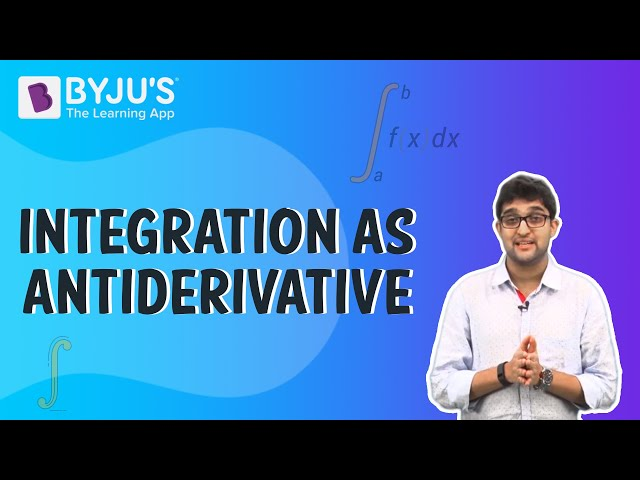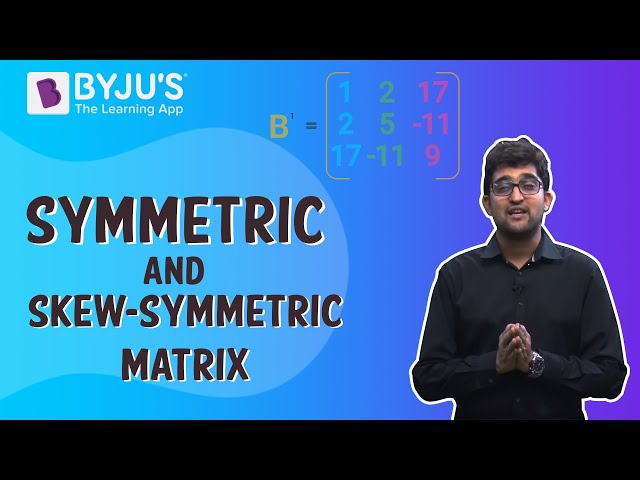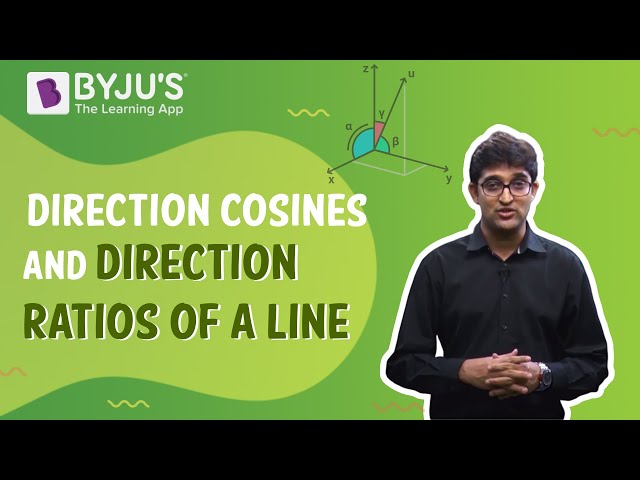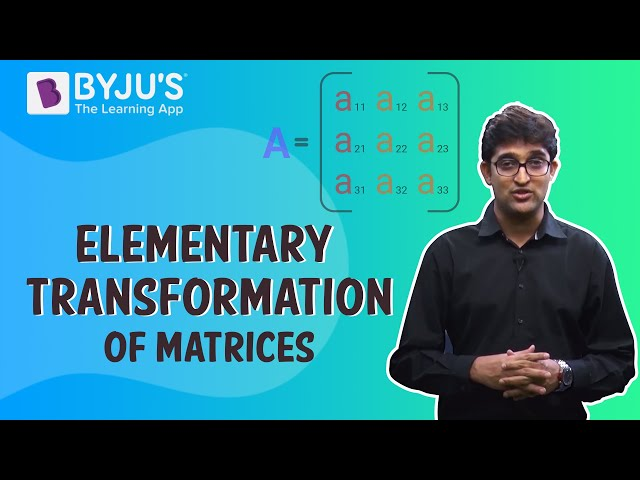# KCET 2015 Mathematics Paper with Solutions

Students can refer to this page to find the solutions for all the problems asked in the KCET 2015 Mathematics paper. These questions are answered by subject experts at BYJU’S and have been prepared in a detailed and easy to understand manner. Aspirant can use the paper to practise solving the questions and develop more efficient problem solving skills required for the examination. Students can also download the question paper with solutions in a PDF format for offline use.
KCET 2015 – Maths

Question 1:

$$\begin{array}{l}f(x)=\frac{1}{2}-tan\frac{\pi x}{2}-1<x< 1\end{array}$$
and
$$\begin{array}{l}g(x)= \sqrt{3+4x-4x^{2}}\end{array}$$
. Find domain of (f+x)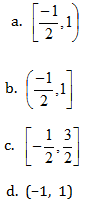$$\begin{array}{l}g(x)= \sqrt{3+4x-4x^{2}}\end{array}$$

Domain of g(x) ⇒ 3+ 4x – 4x2≥0

4x2 – 4x – 3 ≤0

(2x – 1) (2x –3) ≤ 0

x = -1/2, 3/2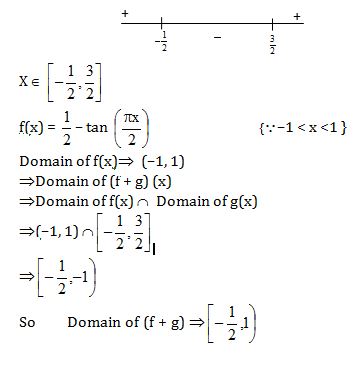Question 2: Write the set builder form A = {–1, 1}

a. A = {x : x is a real number}
b. A = {x : x is an integer}
c. A = {x : x is a root of the equation x2 = 1}
d. A = {x : x is a root of the equation x2 + 1 = 0}

Consider the set A {–1, 1}

Here –1 and 1 are the roots of the equation x2–1 = 0

So set A in set builder form can be written as

A = {x : x is a root of equation x2 – 1 = 0}

Question 3: If the operation ⊕ is defined by a ⊕ b = a2 + b2 for all real number ‘a’ and ‘b’, then (2 ⊕ 3) ⊕ 4 = ________

a. 181
b. 182
c. 184
d. 185

Given that

a ⊕ b = a2 + b2

We have

(2 ⊕3) ⊕4 = (22 + 32) ⊕4

= (4 + 9) ⊕4

= 13 ⊕4 {Using given condition}

= 132 + 42

= 169 + 16

= 185

Question 4: If

$$\begin{array}{l}z=\frac{(\sqrt{3}+i)^{3}(3i+4)^{2}}{(8+6i)^{2}}\end{array}$$
, then
$$\begin{array}{l}\left | z \right |\end{array}$$
is equal to

a. 0
b.1
c. 2
d. 3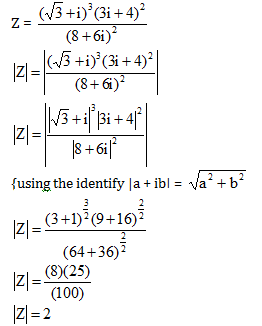Question 5: If α and β are the roots of x2 – ax + b2 = 0, then a2 + b2 = 0, then a2 + b2 is equal to _________

a. a2 –2b2
b. 2a2– b2
c. a2 – b2
d. a2 + b2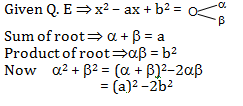Question 6: If the 2nd and 5th term of G.P. are 24 and 3 respectively, then the sum of 1st six terms is ______

a. 189/2
b. 189/5
c. 179/2
d. 2/189

2nd term of G.P ⇒ar = 24 ⇒ a = 24/r …..(1)

5th term of G.P. ⇒ ar4 = 3 …..(2)

Eq. (1) put in Eq (2)

⇒ (24/r). r4 = 3

⇒ 8r3 = 1

r = 1/2

So

a = 24/(1/2) =48

Sum of first six terms is

⇒ a + ar + ar2 + ar3 + ar4 + ar5

⇒ 48 + 24 + 12 + 6 + 3 + (3/2)

⇒ 189/2

Question 7: The middle term of expansion of

$$\begin{array}{l}\left ( \frac{10}{x}+\frac{x}{10} \right )^{10}\end{array}$$

a.7C5
b. 8C5
c. 9C5
d. 10C5

n = 10⇒even

middle terms

$$\begin{array}{l}=\left ( \frac{10}{2}+1 \right )^{th}=6^{th}term\end{array}$$
$$\begin{array}{l}T_{6}=T_{5+1}=^{10}C_{5}\left ( \frac{10}{x} \right )^{5}\left ( \frac{x}{10} \right )^{5}=^{10}C_{5}\end{array}$$

Question 8: If

$$\begin{array}{l}\begin{vmatrix} 2a & x_{1} &y_{1} \\ 2b & x_{2} &y_{2}\\ 2c& x_{3} & y_{3} \end{vmatrix}= \frac{abc}{2}\end{array}$$
not equal to zero, then the area of the triangle whose vertices are
$$\begin{array}{l}\left ( \frac{x_{1}}{a},\frac{y_{1}}{a} \right )\end{array}$$
,
$$\begin{array}{l}\left ( \frac{x_{2}}{b},\frac{y_{2}}{b} \right )\end{array}$$
,
$$\begin{array}{l}\left ( \frac{x_{3}}{c},\frac{y_{3}}{c} \right )\end{array}$$
is

a. (1/4) abc
b. (1/8) abc
c. (1/4)
d. (1/8)

Area of triangle whose vertices are

$$\begin{array}{l}\left ( \frac{x_{1}}{a},\frac{y_{1}}{a} \right )\end{array}$$
,
$$\begin{array}{l}\left ( \frac{x_{2}}{b},\frac{y_{2}}{b} \right )\end{array}$$
,
$$\begin{array}{l}\left ( \frac{x_{3}}{c},\frac{y_{3}}{c} \right )\end{array}$$
is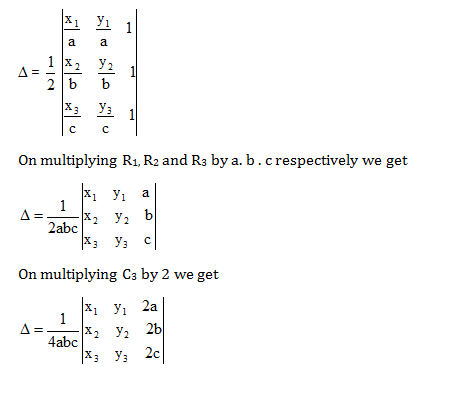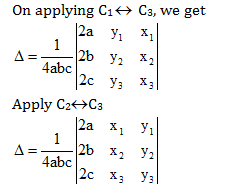= (1/4abc)(abc/2)

= 1/8

Question 9: The shaded region shown in fig. is given by the equation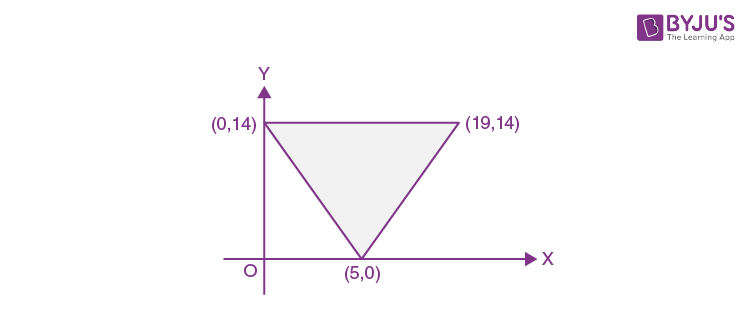a. 14x + 5y ≥70 y ≤14 and x – y ≤ 5
b. 14x + 5y ≥70 y ≤ 14 and x – y ≥5
c. 14x + 5y ≤70 y ≤ 14 and x – y ≥ 5
d. 14x + 5y ≥70 y ≥ 14 and x – y ≥5

Equation of line passing through the coordinate (0, 14) and (9, 14) is

y = 14

Consider the equation of the line joining (0, 14) and (5, 0) applying intercept form gives us

$$\begin{array}{l}\Rightarrow \frac{x}{5}+\frac{y}{14}=1\end{array}$$

⇒14x + 5y = 70

The equation of the line joining (5, 0) and (19, 14) is given by

$$\begin{array}{l}\Rightarrow \frac{y-14}{x-19}=\frac{y-0}{x-5}\end{array}$$

⇒ xy – 14x – 5y + 70 = xy – 19y

x – y = 5

Consider the figure and observe the shaded region the inequalities can be written as

y ≤ 14

14x + 5y ≥ 70

x – y ≤ 5.

Question 10: ~ [(–p) ^q] is logically Equivalent to

a. P ∨ (~q)
b. P ^ (~q)
c. ~ [p^ (~q)]
d. ~ (p^ q)

Question 11: The value of

$$\begin{array}{l}sin^{-1}\left ( \frac{2\sqrt{2}}{3} \right )+sin^{-1}\left ( \frac{1}{3} \right )\end{array}$$

a. π/6
b. π/2
c. π/4
d. 2 π/3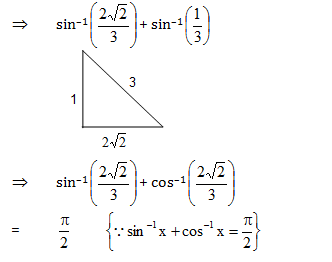Question 12: If the eccentricity of the hyperbola

$$\begin{array}{l}\frac{x^{2}}{a^{2}}-\frac{y^{2}}{b^{2}}=1\end{array}$$
is 5/4 and 2x + 3y – 6 = 0

Is a focal chord of the hyperbola, then the length of transverse axis is equal to

a. 12/5
b. 24/5
c. 6/5
d. 5/24

Given hyperbola has focus (ae, 0) which will lie on 2x + 3y – 6 = 0 as it is focal chord

Therefore, 2ae – 6 = 0

2ae = 6

ae =3 (since e =5/4)

a(5/4) = 3 ; a = (12/5)

Length of transverse axis = 2 a

= 2 × (12/5) = (24/5)

Question 13: If

$$\begin{array}{l}\vec{a}=i+2j+2k\end{array}$$
,
$$\begin{array}{l}\left | \vec{b}\right |=5\end{array}$$
and the angle between
$$\begin{array}{l}\vec{a}=5\end{array}$$
and
$$\begin{array}{l}\vec{b}=5\end{array}$$
is π/6, then the area of the triangle formed by these two vectors as two sides is

a. 15/2
b. 15
c. 15/4
d. 15√3/2

$$\begin{array}{l}\vec{a}=\hat{i}+2\hat{j}+2\hat{k}\end{array}$$
$$\begin{array}{l}\left |a \right |=\sqrt{(1)^{2}+(2)^{2}+(2)^{2}}\end{array}$$
$$\begin{array}{l}\left |a \right |=\sqrt{1+4+4}\end{array}$$
$$\begin{array}{l}\left |a \right |=3\end{array}$$

Required area =

$$\begin{array}{l}\frac{1}{2}\left | a\times b\right |=\frac{1}{2}\times a\times b\times sin\theta\end{array}$$
$$\begin{array}{l}=\frac{1}{2}\times 3\times 5\times sin(\frac{\pi }{6})\end{array}$$
(since θ = π/6)

= 15/4

Question 14: Let

$$\begin{array}{l}\vec{a}=i-2j+3k\end{array}$$
if
$$\begin{array}{l}\vec{b}\end{array}$$
is a vector such that
$$\begin{array}{l}\vec{a}.\vec{b}=\left | \vec{b} \right |^{2}\end{array}$$
and
$$\begin{array}{l}\left | \vec{a}-\vec{b} \right |=\sqrt{7}\end{array}$$
, then
$$\begin{array}{l}\left | \vec{b} \right |=\end{array}$$
—————

a. 7
b. 14
c. √7
d. 21

$$\begin{array}{l}\left | \vec{a}-\vec{b} \right |=\sqrt{7}\end{array}$$

Squaring both side

$$\begin{array}{l}\Rightarrow \left | \vec{a} \right |^{2}+\left | \vec{b} \right |^{2}-2ab =7\end{array}$$
(since
$$\begin{array}{l}\vec{a}.\vec{b}=\left | \vec{b} \right |^{2}\end{array}$$
)

$$\begin{array}{l}\Rightarrow \left [ \sqrt{(1)^{2}+(-2)^{2}+(3)^{2}} \right ]^{2}+\left | \vec{b} \right |^{2}-2\left | \vec{b} \right |^{2}=7\end{array}$$
$$\begin{array}{l}\Rightarrow 14-\left | \vec{b} \right |^{2}=7\end{array}$$
$$\begin{array}{l}\Rightarrow \left | \vec{b} \right |^{2}=7\end{array}$$
$$\begin{array}{l}\Rightarrow \left | \vec{b} \right |=\sqrt{7}\end{array}$$

Question 15: If direction cosines of a vector of magnitude 3 are (2/3), (9/3), (2/3) and a > 0, then vector is _______

a. 2i + j + 2k
b. 2i – j + 2k
c. i –2j + 2 k
d. i + 2j + 2k

Question 16: Equation of line passing through the point (2, 3, 1) and parallel to the line of intersection of the plane x – 2y – z + 5 = 0 and x + y + 3z = 6 is

a.

$$\begin{array}{l}\frac{x-2}{5}=\frac{y-3}{-4}=\frac{z-1}{3}\end{array}$$

b.
$$\begin{array}{l}\frac{x-2}{-5}=\frac{y-3}{-4}=\frac{z-1}{3}\end{array}$$

c.
$$\begin{array}{l}\frac{x-2}{5}=\frac{y-3}{4}=\frac{z-1}{3}\end{array}$$

d.
$$\begin{array}{l}\frac{x-2}{4}=\frac{y-3}{3}=\frac{z-1}{2}\end{array}$$

Consider the planes

X – 2y – Z + 5 = 0 and x + y + 3z = 6

Let the DR’s of the line passing through the intersection of the given plane be a, b and C

a– 2b – C = 0 and a + b + 3c = 0

Thus

$$\begin{array}{l}\frac{a}{-6+1}=\frac{-b}{3+1}=\frac{C}{1+2}\end{array}$$
$$\begin{array}{l}\frac{a}{-5}=\frac{-b}{4}=\frac{C}{3}\end{array}$$

Now the required line passes through (2, 3, 1) and is parallel to the above line.

The equation of the required line is,

$$\begin{array}{l}\frac{x-2}{-5}=\frac{y-3}{-4}=\frac{z-1}{3}\end{array}$$

Question 17: Foot of perpendicular drawn from the origin to the plane 2x – 3y + 4z = 29 is _______

a. (5, –1, 4)
b. (2, –3, 4)
c. (7, –1, 3)
d. (5, –2, 3)

Consider the equation of plane,

2x – 3 y + 4z = 29

The DR’s of the line perpendicular to line form origin is 2, – 3 and 4

The equation of the line will be

$$\begin{array}{l}\frac{x-0}{2}=\frac{y-0}{-3}=\frac{z-0}{4}=\lambda\end{array}$$

So the coordinates of the foot is (2 λ, –3 λ, 4 λ)

Since the foot lies on the plane, hence

4 λ+9 λ + 16 λ = 29

29 λ = 29

29 λ= 1

Thus, the coordinate of the foot are (2, –3, 4)

Question 18: If two dice are thrown simultaneously, then the probability that the sum of the number which comes up on the dice to be more than 5 is ___________.

a. 5/36
b. 1/6
c. 5/18
d. 13/18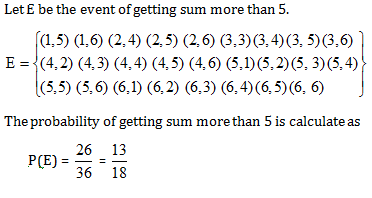Question 19: If y= f(x2+2) and f’(3) =5, then dy/dx at x =1 is ___________.

a. 5
b. 25
c. 15
d. 10

y = f (x2 + 2)

Differentiate w.r.t x

(dy/dx) = f (x2 + 2). 2x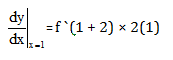= f (3) × 2

= 2(5) = 10.

Question 20: If x = a cos3θ, y = a sin3θ, then 1+ (dy/dx)2 is ___________.>

a. tan θ
b. tan2 θ
c. Sec2 θ
d. 1

Consider the expression.

X = acos3θ

Differentiate w.r.t θ

(dx/d θ) = –3acos2 θ. sin θ ……(1)

and y = asin3 θ

Differentiate w.r.t θ

(dy/d θ)= 3asin2 θ. cos θ ……..(2)

Divide equation (2) by (1)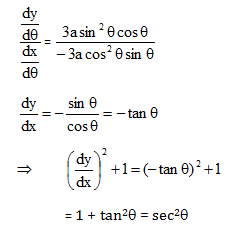Question 21: Slope of Normal to the curve

$$\begin{array}{l}y = x^{2}-\frac{1}{x^{2}}\end{array}$$
at (-1,0) is

a. 1/4
b. -1/4
c. 4
d. –4

$$\begin{array}{l}y = x^{2}-\frac{1}{x^{2}}\end{array}$$

Differentiate w.r.t x

$$\begin{array}{l}\frac{dy}{dx}=2x+\frac{2}{x^{3}}\end{array}$$
$$\begin{array}{l}\left.\frac{d y}{d x}\right|_{x-1}=2(-1)+\frac{2}{(-1)^{3}}\end{array}$$

= – 2 – 2 = –4

$$\begin{array}{l}Slope =\frac{-1}{\frac{dy}{dx}}=\frac{-1}{-4}=\frac{1}{4}\end{array}$$

Question 22:

$$\begin{array}{l}\int \frac{1}{x^{2}(x^{4}+1)\frac{3}{4}}dx\end{array}$$
is equal to ___________.

a.

$$\begin{array}{l}\frac{-(1+x^{4})^{1/4}}{x}+C\end{array}$$

b.
$$\begin{array}{l}\frac{-(1+x^{4})^{1/4}}{x^{2}}+C\end{array}$$

c.
$$\begin{array}{l}\frac{-(1+x^{4})^{1/4}}{2x}+C\end{array}$$

d.
$$\begin{array}{l}\frac{-(1+x^{4})^{3/4}}{x}+C\end{array}$$

Let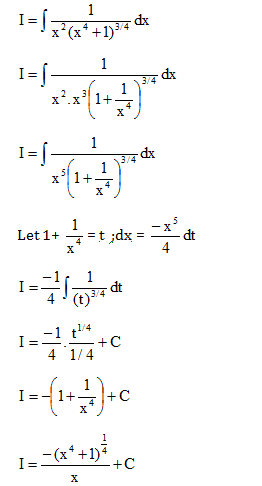Question 23: If f : R → R is defined by f(x) = x/(x 2+2), find f(f(2))

a. 1/29
b. 10/29
c. 29/10
d.29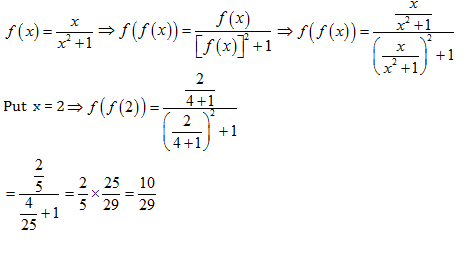Question 24: Evaluate

$$\begin{array}{l}\begin{vmatrix} cos 15 & sin 15 \\ sin 75& cos 75 \end{vmatrix}\end{array}$$

a. 1
b. 0
c. 2
d. 3

$$\begin{array}{l}\begin{vmatrix} cos 15^{0} & sin 15^{0} \\ sin 75^{0}& cos 75^{0} \end{vmatrix}[\end{array}$$

= cos 15° cos 75° – sin 15° sin 75°

= cos (15 + 75)

= cos 90°

= 0

Question 25: A man takes a step forward with probability 0.4 and one step backward with probability 0.6 then the probability that at the end of eleven steps he is one step away from the starting point is

a. 11C5× (0.48)5
b. 11C6× (0.24)5
c. 11C5× (0.12)5
d. 11C6× (0.72)6

One step away means a step forward or a step backward either he took 6 forward steps and 5 backward steps or 6 backward steps and 5 forward steps

⇒required probability

= 11C6(0.4)6(0.6)5 + 11C5(0.4)5(0.6)6 = 11C5(0.4)5(0.6)5[0.4+0.6]= 11C5(0.24)5= 11C6× (0.24)5

Question 26:.

$$\begin{array}{l}\int_{0}^{\pi /4}log\left ( \frac{sin x+cos x}{cosx} \right )dx\end{array}$$

a. (π/4) log2
b. (π/2) log2
c. (π/8) log2
d. Log2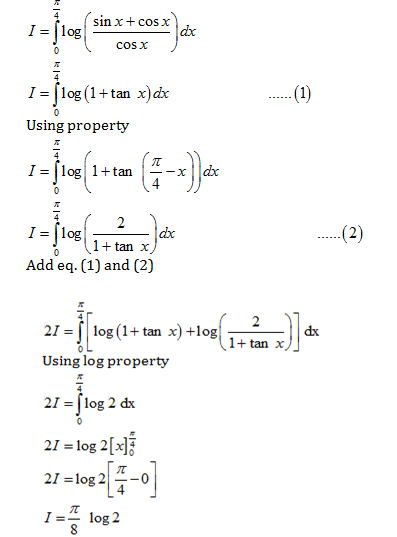Question 27: Area bounded by y = x3, y = 8 and x = 0 is _______

a. 2 sq. unit
b. 4 sq. unit
c. 12 sq. unit
d. 6 sq. unit

Consider the graph of y = x3 and y = 8 on the same plane.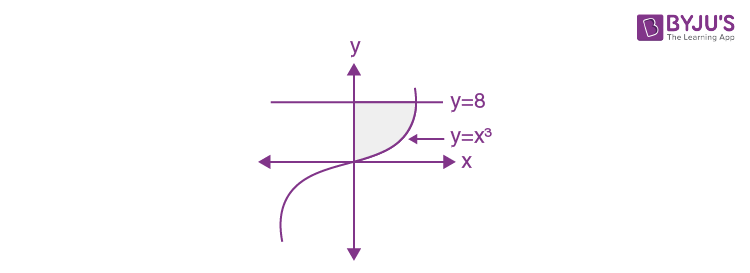The required area is the shaded region.

$$\begin{array}{l}A=\int_{0}^{A}y^{1/3}dy\end{array}$$

A = (3/4)(16)

A=12 sq.units

Question 28: Let

$$\begin{array}{l}\vec{a}=i+2j+k\end{array}$$
,
$$\begin{array}{l}\vec{b}=i-j+k\end{array}$$
and
$$\begin{array}{l}\vec{c}=i+j-k\end{array}$$
, a vector in the plane
$$\begin{array}{l}\vec{a}\end{array}$$
and whose projection on is is ______________.

a. 3i + j – 3k
b. 4i + j – 4k
c. I+ j – 2k
d. 4i – j + 4k

Given vectors

$$\begin{array}{l}\vec{a}=i+2j+k\end{array}$$
$$\begin{array}{l}\vec{b}=i-j+k\end{array}$$
$$\begin{array}{l}\vec{c}=i+j-k\end{array}$$

The vector r in the plane a and b is calculated as

$$\begin{array}{l}\vec{r}=\vec{a}+\lambda \vec{b}\end{array}$$
$$\begin{array}{l}\vec{r}= (1+\lambda )\hat{i}+2(1-\lambda )\hat{i}+(1+\lambda )\hat{k}\end{array}$$
——–(1)

$$\begin{array}{l}Projection =\frac{\vec{r}\vec{c}}{\left | \vec{c} \right |}\end{array}$$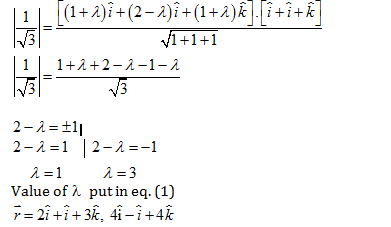Question 29: The mean deviation from the data 3, 10, 10, 4, 7, 10, 5:

a. 3
b. 2
c. 3. 75
d. 2.75

$$\begin{array}{l}Mean = \frac{3+4+5+7+10+10+10}{7}\end{array}$$

= 49/7

=7

Median =

$$\begin{array}{l}\left ( \frac{7+1}{2} \right )^{th}\end{array}$$
observation

= 4th observation

Median = 7

Mean deviation =

$$\begin{array}{l}\frac{1}{7}\left [ \left | 7-3 \right |+\left | 7-4 \right |+\left | 7-5 \right |+\left | 7-7 \right |+\left | 7-10 \right |+\left | 7-10 \right |+\left | 7-10 \right | \right ]\end{array}$$

= (1/7) (4+3+2+0+3+3+3)

= 18/7 = 2.57

Question 30: The probability distribution of x is

 X 0 1 2 3 P(x) 0.2 K K 2k

Find the value of k

a. 0.2
b. 0.3
c. 0.4
d. 0.1

$$\begin{array}{l}\sum P(x)=1\end{array}$$

0.2 +k +k +2k =1

0.2 +4k =1

4k =0.8

K=0.2

Question 31: If the function g(x) is defined by

$$\begin{array}{l}g(x)=\frac{x^{200}}{200}+\frac{x^{199}}{199}+\frac{x^{198}}{198}+——+\frac{x^{2}}{2}+x+5\end{array}$$

a. 1
b. 200
c. 100
d. 5

$$\begin{array}{l}g(x)=\frac{x^{200}}{200}+\frac{x^{199}}{199}+\frac{x^{198}}{198}+…….+\frac{x^{2}}{2}+x+5\end{array}$$

Differentiate w.r.t x

$$\begin{array}{l}g'(x)=\frac{200x^{199}}{200}+\frac{199x^{198}}{199}+\frac{198x^{197}}{198}+…….+\frac{2x}{2}+1\end{array}$$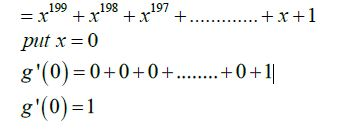Question 32: A box contains 6 red marbles numbers from 1 through 6 and 4 white marbles 12 through 15 Find the probability that a marble drawn ‘at random’ is white and odd numbered.

a. 5
b. 1/5
c. 6
d. 1/6

The total number of marbles are n(S) = 6 + 4 = 10

The number of marbles that are white and odd numbered is n(E) = 2

$$\begin{array}{l}Probability=\frac{n(E)}{n(S)}=\frac{2}{10}=\frac{1}{5}\end{array}$$

Question 33:

$$\begin{array}{l}\lim_{x\rightarrow 0}\frac{1-cosx}{x^{2}}\end{array}$$
is ______________.

a. 2
b. 3
c. (1/2)
d. (1/3)

$$\begin{array}{l}\lim_{x\rightarrow 0}\frac{1-cosx}{x^{2}}\end{array}$$
(
$$\begin{array}{l}\frac{0}{0} form\end{array}$$
)

Using L’ Hospital rule

$$\begin{array}{l}\Rightarrow \lim_{x\rightarrow 0}\frac{sinx}{2x}\end{array}$$
$$\begin{array}{l}\Rightarrow\frac{1}{2} \lim_{x\rightarrow 0}\frac{sinx}{2x}\end{array}$$

= (1/2)(1)

= ½

Question 34:

$$\begin{array}{l}f(x)=\left\{\begin{array}{ll} 3 x-8 & \text { if } x \leq 5 \\ 2 k & \text { if } x>5 \end{array}\right.\end{array}$$
is continuous, find k.

a. 2/7
b. 3/7
c. 4/7
d. 7/2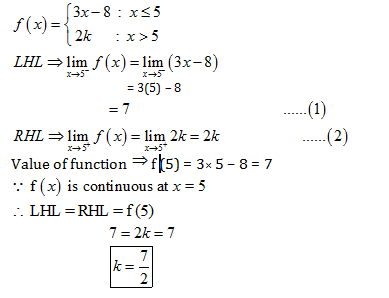Question 35: If f(x) = 2x2, find

$$\begin{array}{l}\frac{f(3.8)-f(4)}{3.8-4}\end{array}$$
Choose the correct option:

a. 1.56
b. 156
c. 15.6
d. 0.156

$$\begin{array}{l}\frac{f(3.8)-f(4)}{3.8-4}=\frac{2(3.8)^{2}-2(4)^{2}}{3.8-4}\end{array}$$

= (28.88 -32)/-0.2

= -3.12/-0.2

= 15.6

Question 36: If x = ct and y =c/t , find dy/dx at t = 2.

a. 1/4
b. 4
c. -1/4
d.0

x=ct

Differentiate w.r.t t

$$\begin{array}{l}\frac{dx}{dt}=c\end{array}$$
———-(1)

And

$$\begin{array}{l}y=\frac{c}{t}\end{array}$$

Differentiate w.r.t t

$$\begin{array}{l}\frac{dy}{dt}=-\frac{c}{t^{2}}\end{array}$$
——(2)

Divide equation (2) by (1)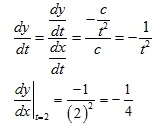Question 37: A balloon which always remains spherical is being inflated by pumping in 10 cubic centimeters of gas per second. Find the rate at which the radius of the balloon is increasing when the radius is 15 cms

a. (1/90 π) cm/sec
b. (1/9 π) cm/sec
c. (1/30 π) cm/sec
d. (1/π) cm/sec

The rate of pumping the gas in the balloon is (dv/dt) = 10cm3/sec

The volume of the spherical balloon is given by v= (4/3) πr3 differentiate w.r.t r

(dv/dr) = (4/3) π(3r2)

= 4 πr2

$$\begin{array}{l}\left.\frac{d v}{d r}\right|_{r-15}=4 \pi(15)^{2}=900 \pi\end{array}$$

Now,

$$\begin{array}{l}\frac{dv}{dt}=\frac{dv}{dr}\times \frac{dr}{dt}\end{array}$$
$$\begin{array}{l}10=900\pi \times \left ( \frac{dr}{dt} \right )\end{array}$$
$$\begin{array}{l}\frac{dr}{dt}=\frac{1}{90}\pi cm/sec\end{array}$$

Question 38:

$$\begin{array}{l}\int \frac{sin^{2}x}{1+cos x}dx\end{array}$$

a. x + sinx + C
b. x – sinx + C
c. Sin x + C
d. Cos x + C

$$\begin{array}{l}I=\int \frac{\sin ^{2} x}{1+\cos x} d x\end{array}$$
$$\begin{array}{l}I=\int \frac{\left(1-\cos ^{2} x\right)}{1+\cos x} d x\end{array}$$
$$\begin{array}{l}I=\int \frac{(1-\cos x)(1+\cos x)}{(1+\cos x)} d x\end{array}$$
$$\begin{array}{l}I=\int(1-\cos x) d x\end{array}$$
$$\begin{array}{l}I=x-\sin x+c\end{array}$$

Question 39:

$$\begin{array}{l}\int e^{x}\left [\frac{1+sinx}{1+cos x} \right ]dx\end{array}$$

a.

$$\begin{array}{l}e^{x}tan(\frac{x}{2})+C\end{array}$$

b.
$$\begin{array}{l}tan(\frac{x}{2})+C\end{array}$$

c.
$$\begin{array}{l}e^{x}+C\end{array}$$

d.
$$\begin{array}{l}e^{x}sin x+C\end{array}$$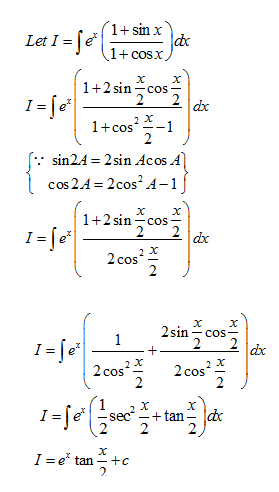Question 40: If 1, w, w2 are three cube roots of unity, then (1–w + w2) (1 + w – w2) is __________

a.1
b.2
c. 3
d. 4

If 1, w, w2 are three cube roots of unity,

then 1 + w + w2 = 0 ……..(1)

Hence

(1-w + w2) (1 + w – w2) = (1 + w + w2 – 2w) (1+ w + w2 – 2w2)

= (0 – 2 w) (0 – 2w2)

{from eq. (1)}

= ( – 2 w) ( – 2w2)

= 4w3

= 4(1)

= 4

Question 41: Solve for x

$$\begin{array}{l}tan^{-1}\left ( \frac{1-x}{1+x} \right )=\frac{1}{2}tan^{-1}x,x> 0\end{array}$$

a. √3
b. 1
c. –1
d. 1/√3

$$\begin{array}{l}\tan ^{-1}\left(\frac{1-x}{1+x}\right)=\frac{1}{2} \tan ^{-1} x\end{array}$$
$$\begin{array}{l}\tan ^{-1}(1)-\tan ^{-1} x=\frac{1}{2} \tan ^{-1} x \Rightarrow \tan ^{-1} x+\frac{1}{2} \tan ^{-1} x=\tan ^{-1}(1)\end{array}$$
$$\begin{array}{l}\frac{3}{2} \tan ^{-1} x=\tan ^{-1}(1) \Rightarrow \frac{3}{2} \tan ^{-1} x=\frac{\pi}{4}\end{array}$$
$$\begin{array}{l}\tan ^{-1} x=\frac{\pi}{6}\end{array}$$
$$\begin{array}{l}x=\tan \frac{\pi}{6}\end{array}$$
$$\begin{array}{l}x=\frac{1}{\sqrt{3}}\end{array}$$

Question 42: The system of linear equation x + y + z = 6, x + 2y + 3z = 10 and x + 2y + az = 6 has no solutions when __________

a. a = 2, b ≠ 3
b. a = 3, b ≠ 10
c. b = 2, a = 3
d. b = 3, a ≠ 3

Consider the given system of equations

x + y + z = 6 ……….(1)

x + 2y + 3z = 10 ……….(2)

x + 2y + az = 6 ……….(3)

Since condition of no solution, Δ=0

So,

$$\begin{array}{l}\begin{vmatrix} 1 & 1 &1 \\ 1 & 2 &3 \\ 1& 2 &a \end{vmatrix}=0\end{array}$$

⇒ 1(2a-6)-1(a-3)+1(2-2)=0

⇒ 2a -6-a+3 =0

⇒ a=3

Now, b ≠ 10 then equations (2) and (3) will become identical, which will have infinite solutions.

The condition is satisfied for the option a = 3 and b ≠ 10.

Question 43: The value of tan (10) + tan (890) is ___________

a. 1/sin 10
b. 2/sin 20
c. 2/sin 10
d. 2/sin 20

⇒ tan (10) + tan (890)

⇒ tan (10) + tan (900-10)

⇒ tan (10) + cot (10) =

$$\begin{array}{l}\frac{sin 1^{0}}{cos1^{0}}+\frac{cos1^{0}}{sin1^{0}}\end{array}$$
$$\begin{array}{l}=\frac{sin^{2} 1^{0}+cos^{2}1^{0}}{sin(1^{0})cos(1^{0})}=\frac{1}{sin(1^{0})cos(1^{0})}\end{array}$$
$$\begin{array}{l}=\frac{2}{2sin(1^{0})cos(1^{0})}=\frac{2}{sin(2^{0})}\end{array}$$

Question 44:

$$\begin{array}{l}\frac{(x+1)^{2}}{x^{3}+x}=\frac{A}{x}+\frac{Bx+C}{x^{2}+1}\end{array}$$
, then cosec-1 (1/A) + cot-1(1/B) +sec-1C=___________

a. 5π/6
b. 0
c. 5π/6
d. π/2

Consider the expression

$$\begin{array}{l}cosec^{-1}\frac{1}{A}+cot^{-1}\frac{1}{B}+sec^{-1}c\end{array}$$

Also,

$$\begin{array}{l}\frac{x^{2}+2x+1}{x(x^{2}+1)}=\frac{A}{x}+\frac{Bx+C}{x^{2}+1}\end{array}$$
$$\begin{array}{l}\frac{1}{x}+\frac{2}{x^{2}+1}=\frac{A}{x}+\frac{Bx+C}{x^{2}+1}\end{array}$$

Compare the like terms of the equation

A = 1

B = 0

C = 2

Value of A, B, C put in equation (1)

$$\begin{array}{l}cosec^{-1}\left ( \frac{1}{1} \right )+cot^{-1}(\frac{1}{0})+sec^{-1}(2)\Rightarrow \frac{\pi }{2}+cot^{-1}(\infty )+\frac{\pi }{3}\end{array}$$

= (5 π/6) + 0 = 5 π/6

Question 45: The remainder obtained when 1! + 2! + 3! + ……+ 11! Is divided by 12 is ________

a. 9
b. 8
c. 7
d. 6

Given:1! 2!+3!+4!+—– +11!

=(1!+2!+3!)+(4!+5!+6!+—–+11!)

=(1+2+6) + (4!+5!+6!+—-+11!)

=9+(4!+5!+6!+—-+11!)

4!, 5!, 6! —– 11! Are divisible by 12 so, when you will divide given expression by 12 the remainder be 9.

Question 46: If α ≤2sin–1 x + cos–1 x ≤ β, then

a. α = – π/2 , β = π/2
b. α = – π/2 , β = 3π/2
c. α = 0, β = π
d. α = 0, β =2 π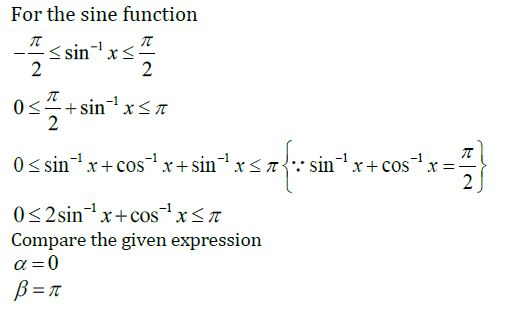Question 47: If A =

$$\begin{array}{l}\begin{bmatrix} 0 &1 \\ 1 & 0 \end{bmatrix}\end{array}$$
, then A2 equal to _________.

a.

$$\begin{array}{l}\begin{bmatrix} 0 &1 \\ 1 & 0 \end{bmatrix}\end{array}$$

b.
$$\begin{array}{l}\begin{bmatrix} 1 &0 \\ 1 & 0 \end{bmatrix}\end{array}$$

c.
$$\begin{array}{l}\begin{bmatrix} 1 &0 \\ 0 & 1 \end{bmatrix}\end{array}$$

d.
$$\begin{array}{l}\begin{bmatrix} 0 &1 \\ 0 & 1 \end{bmatrix}\end{array}$$

A =

$$\begin{array}{l}\begin{bmatrix} 0 &1 \\ 1 & 0 \end{bmatrix}\end{array}$$

A2=A.A

$$\begin{array}{l}= \begin{bmatrix} 0 &1 \\ 1 & 0 \end{bmatrix}\begin{bmatrix} 0 &1 \\ 1 & 0 \end{bmatrix}\end{array}$$

=

$$\begin{array}{l}\begin{bmatrix} 0+1 &0+0 \\ 0+0 & 1+0 \end{bmatrix}\end{array}$$

=

$$\begin{array}{l}\begin{bmatrix} 1 &0 \\ 0 & 1 \end{bmatrix}\end{array}$$

Question 48: The function f(x) = [x], where [x] denotes greatest integer function is continuous at _________

a. 4
b. –2
c. 1
d. 1.5

Consider the function

F(x) = [x]

Here, [x] denotes the greatest integer function.

[x] is always continuous at non integer value of x.

Thus, the function will be continuous at x=1.5

Question 49: If

$$\begin{array}{l}y = log\left ( \frac{1-x^{2}}{1+x^{2}} \right )\end{array}$$
, then (dy/dx) is equal to _________

a.

$$\begin{array}{l}\frac{-4x}{1-x^{4}}\end{array}$$

b. Stem height
c.
$$\begin{array}{l}\frac{1}{4-x^{4}}\end{array}$$

d.
$$\begin{array}{l}\frac{-4x^{3}}{1-x^{4}}\end{array}$$

$$\begin{array}{l}Y =log\left ( \frac{1-x^{2}}{1+x^{2}} \right )\end{array}$$

using log property

y=log(1-x2)-log(1+x2)

differentiate w.r.t x

$$\begin{array}{l}\frac{dy}{dx}=\frac{1}{(1-x^{2})}(-2x)-\frac{1}{(1+x^{2})}(2x)\end{array}$$
$$\begin{array}{l}\frac{dy}{dx}=(-2x)\left [\frac{1}{(1-x^{2})}+\frac{1}{(1+x^{2})} \right ]\end{array}$$
$$\begin{array}{l}\frac{dy}{dx}=(-2x)\left [\frac{1+x^{2}+1-x^{2}}{(1-x^{2})(1+x^{2})} \right ]\end{array}$$
{since,(A-B)(A+B)= A2-B2}

= -4x/(1-x4)

Question 50: The two curves x3 –3xy2 + 2 = 0 and 3x2y – y3 = 2

a. Touch each other
b. Cut at right angle
c. Cut at angle π/3
d. Cut at angle π/4

First curve equation

x3-3xy2+2=0

Differentiate w.r.t. x

3x2-3(y2+2xy y’)=0

3x2-3y2-6xy y’)=0

y’=

$$\begin{array}{l}\frac{3x^{2}-3y^{2}}{6xy}=m_{1}\end{array}$$

second curve equation

3x2y-y3= 2

Differentiate w.r.t x

⇒ 3(2xy + x2y’)-3y2y’= 0

⇒ 6xy + 3x2y’-3y2y’= 0

$$\begin{array}{l}y^{1}=\frac{-6xy}{3x^{2}-3y^{2}}=m_{2}\end{array}$$

Calculate the product of slope

$$\begin{array}{l}m_{1}\times m_{2}=\left (\frac{-6xy}{3x^{2}-3y^{2}} \right )\left ( \frac{3x^{2}-3y^{2}}{6xy} \right )\end{array}$$

m1 × m2 = -1

The product is -1. So the curves intersect at each other at right angle.

Question 51: If x is real, then the minimum value of x2 – 8x + 17 is ___________

a. 1
b. 2
c. 3
d. 4

Let

y=x2-8x+17

y=(x2-8x+16)+1

y=(x-4)2+1

so, y≥1 for all real values of x as (x-4)2≥0

Thus, the minimum value of y is 1

Question 52:

$$\begin{array}{l}\int_{-\frac{\pi }{2}}^{\frac{\pi }{4}}\frac{dx}{1+cos2x}\end{array}$$
is equal to

a. 2
b.1
c. 4
d. 0

Let

$$\begin{array}{l}I=\int_{\frac{\pi}{4}}^{\frac{\pi}{4}} \frac{d x}{1+\cos 2 x}\end{array}$$
$$\begin{array}{l}I=\int_{\frac{\pi}{4}}^{\frac{\pi}{4}} \frac{d x}{1+2\cos ^{2} x-1}\end{array}$$
$$\begin{array}{l}I=\int_{\frac{\pi}{4}}^{\frac{\pi}{4}} \frac{1}{2}sec^{2}x\end{array}$$

Let

f(x) = sec2x

f(-x)=sec2(-x)

f(-x)=sec2x

f(-x)=f(x)

so f(x) is an even function

Therefore,

$$\begin{array}{l}I=2\int_{0}^{\frac{\pi}{4}} \frac{1}{2}sec^{2}xdx\end{array}$$
$$\begin{array}{l}I=\int_{0}^{\frac{\pi}{4}} sec^{2}xdx\end{array}$$
$$\begin{array}{l}I=[\tan x]_{0}^{\pi / 4}\end{array}$$
$$\begin{array}{l}I=\tan \frac{\pi}{4}-\tan 0\end{array}$$

I = 1-0

I = 1

Question 53: The order of differential equation of all circles of given radius ‘a’ is _________

a. 4
b. 2
c. 1
d. 3

Let the centre of circle be (h,k) and radius be r.

The equation of circle can now be written as,

(x-h)2+(y-k)2 = x2 …..(1)

Hence the differential equation is of order 2.

Question 54: The solution of differential equation

$$\begin{array}{l}x\frac{dy}{dx}+2y=x^{2}\end{array}$$
is

a.

$$\begin{array}{l}y=\frac{x^{2}+c}{4x^{2}}\end{array}$$

b.
$$\begin{array}{l}y=\frac{x^{2}}{4}\end{array}$$

c.
$$\begin{array}{l}y=\frac{x^{4}+c}{x^{2}}\end{array}$$

d.
$$\begin{array}{l}y=\frac{x^{4}+c}{4x^{2}}\end{array}$$

$$\begin{array}{l}x\frac{dy}{dx}+2y=x^{2}\end{array}$$
$$\begin{array}{l}\frac{dy}{dx}+\frac{2}{x}y=x\end{array}$$

The above equation is a linear equation in terms of y.

Therefore,

I.F =

$$\begin{array}{l}e^{\int \frac{2}{x}dx}\end{array}$$
$$\begin{array}{l}= e^{2log x}\end{array}$$
$$\begin{array}{l}= e^{log x^{2}}\end{array}$$

=x2

The required solution is

$$\begin{array}{l}y.x^{2}=\int (x.x^{2})dx+c_{1}\end{array}$$
$$\begin{array}{l}yx^{2}= \frac{x^{4}}{4}+c_{1}\end{array}$$
$$\begin{array}{l}y=\frac{x^{4}+4c_{1}}{4x^{2}}\end{array}$$
$$\begin{array}{l}y=\frac{x^{4}+c}{4x^{2}}\end{array}$$

Question 55: If sin x + sin y =(1/2) and cos x + cos y = 1, then tan (x + y) = ___________

a. 8/3
b. -3/4
c. -8/3
d. 4/3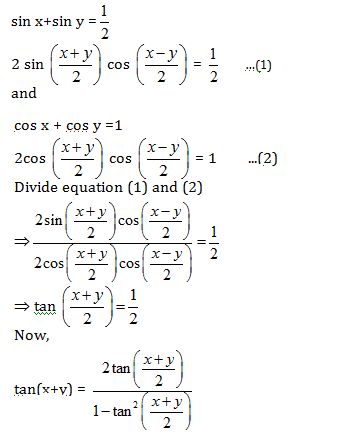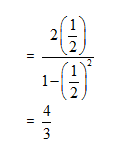Question 56: If

$$\begin{array}{l}A=\begin{bmatrix} \alpha & 2\\ 2& \alpha \end{bmatrix}\end{array}$$
and
$$\begin{array}{l}\left | A^{3} \right |=27\end{array}$$
, then α =___________

a. ±1
b. ±2
c. ±√7
d. ±√5

Given

$$\begin{array}{l}A=\begin{bmatrix} \alpha & 2\\ 2 & \alpha \end{bmatrix}\end{array}$$
$$\begin{array}{l}\left | A \right |=\begin{vmatrix} \alpha & 2\\ 2& \alpha \end{vmatrix}\end{array}$$
$$\begin{array}{l}\left | A \right |=\alpha ^{2}-4\end{array}$$

Now,

$$\begin{array}{l}\left | A ^{3}\right |=27\end{array}$$
$$\begin{array}{l}(\alpha ^{2}-4)^{3}=3^{3}\end{array}$$
$$\begin{array}{l}(\alpha ^{2}-4)=3\end{array}$$
$$\begin{array}{l}\alpha ^{2}=7\end{array}$$

So,

$$\begin{array}{l}\alpha =\pm \sqrt{7}\end{array}$$

Question 57: If

$$\begin{array}{l}P= \begin{vmatrix} x & 1\\ 1 & x \end{vmatrix}\end{array}$$
and
$$\begin{array}{l}\begin{vmatrix} x & 1 & 1\\ 1 & x & 1\\ 1 & 1 & x \end{vmatrix}\end{array}$$
, then dQ/dx = =___________

a. 3p +1
b. 1–3p
c. –3p
d. 3p

$$\begin{array}{l}P= \begin{vmatrix} x & 1\\ 1 & x \end{vmatrix}\end{array}$$
$$\begin{array}{l}\left | P \right |= x^{2}-1\end{array}$$

And

$$\begin{array}{l}\theta =\begin{vmatrix} x & 1 & 1\\ 1 & x & 1\\ 1 & 1 & x \end{vmatrix}\end{array}$$

θ= x(x2-1)-1(x-1)+1(1-x)

θ= x3-x-x+1+1-x

θ= x3-3x+2

d.fferentiate w.r.t x

$$\begin{array}{l}\frac{d\theta }{dx}=3x^{2}-3\end{array}$$

= 3(x2-1)

= 3P

Question 58: A line passes through (2, 2) and is perpendicular in the line 3x + y = 3 its y-intercept is ____

a. 1/3
b. 2/3
c. 4/3
d. 1

The equation of line is 3x+y = 3

slope of the line ⇒

$$\begin{array}{l}3+\frac{dy}{dx}=0\end{array}$$
$$\begin{array}{l}\Rightarrow \frac{dy}{dx}=-3=m\end{array}$$

slope of the perpendicular line ⇒

$$\begin{array}{l}\frac{-1}{m}=\frac{-1}{-3}=\frac{1}{3}\end{array}$$

The equation of line passing through (2,2) and perpendicular to given line

⇒ (y-2) =(1/3)(x-2)

3y-x = 4

$$\begin{array}{l}\frac{3y}{4}-\frac{x}{4}=1\end{array}$$

Thus, the y-intercept is (4/3)

Question 59: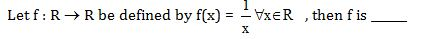a. One-one
b. onto
c. bijective
d. f is not defined

c.nsider the function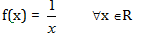substitute 0 for x in the above expression

f(x) = 1/0

= undefined

Thus, the function f(x) is not defined

Question 60: The solution set of the inequation

$$\begin{array}{l}\frac{x^{2}+6x-7}{\left | x+4 \right |}< 0\end{array}$$
is ___________

a. (-7,1)
b. (-7,-4)
c.

$$\begin{array}{l}(-7,-4)\cup (-4,1)\end{array}$$

d.
$$\begin{array}{l}(-7,-4)\cup (4,1)\end{array}$$

$$\begin{array}{l}\frac{x^{2}+6x-7}{\left | x+4 \right |}< 0\end{array}$$

for the above expression

$$\begin{array}{l}\left | x+4 \right |> 0\end{array}$$

a.d

x2+6x-7<0 { x ≠ -4 }

(x+7)(x-1)<0

Diagram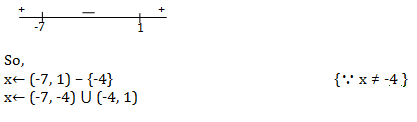## Video Lessons – KCET 2015 – Maths